# Dielectric behavior law: definitions

## Definition

We call the dielectric behavior law the macroscopic relationship that connects the electric polarization P to the local electric field strength E in any point of the material.

This law means that the electric polarization of the material is modified under the effect of the electric field.

This law can be written in the form: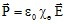where the quantity χ e is a scalar or a tensor quantity called electric susceptibility*.

Attention: * the quantity χe depends on E; thus, χe must be written in the form χe(E) in the previous relationship.

## Note

The dielectric behavior law can be written in one of the following forms: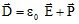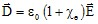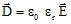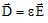where the quantity ε is the scalar or the tensor of the permittivity.

Attention: * the quantities χe, εr, ε must be written in the form χe(E), εr(E), ε(E) in all the previous relationships.

## Complex permittivity

In the case of a harmonic regime, the complex representation is used.

The permittivity is expressed by the complex quantity: ε = ε' - j.ε "

where:

• ε' is the real part of the complex permittivity ε

( ε' is the term that characterizes the velocity of the wave inside the material: the bigger ε' is, the smaller the velocity)

• ε" is the imaginary part of the complex permittivity ε

( ε" is the term that characterizes the dielectric losses in the material, i.e. the capacity of the material to transform the electromagnetic energy into thermal energy)

The dielectric losses* are characterized by the quantity tan( δh )= ε "/ ε'

(where δh is the dielectric loss angle by hysteresis)

Note: Complementary information on dielectric losses can be found in chapter Electric applications: principles (see § Ohmic and dielectric losses ).